## The `Mod` Operator

In this lab, you will program procedures to carry out mathematical tasks.

On this page, you create a predicate to check whether one number is divisible by another.Katherine Johnson (1918–2020) was an aerospace technologist with a Ph.D. in mathematics who worked for NASA calculating the motion of spacecraft. Johnson, originally hired as a human computer, verified the calculations of digital computers, helped calculate the trajectory of Apollo 11 (the first time humans walked on the Moon), worked on plans for a mission to Mars, and encouraged students to pursue careers in science, technology, engineering, and mathematics.

NASA's memorial video: "Katherine Johnson: An American Hero"

1. "U2L4-MathLibrary"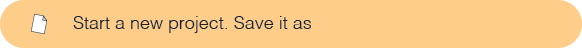2. Experiment with the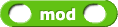block.
1. Try various inputs.
2. Keep the second number constant, and try various inputs for the first number.
3. Form a hypothesis. What do you notice?
The `mod` block reports the remainder when the first input is divided by the second. For example,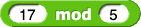reports 2 because when 17 is divided by 5, the remainder is 2. When one number divides another evenly, the remainder is 0. So for example,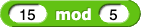reports 0.
Different programming languages have different ways of handling negative inputs to the mod function. So you won't see any negative numbers used with mod on the exam.
The `mod` operator: The expressionwould be written as
`17 MOD 5`
on the exam. If you see an expression with variables as input to `mod`, such as
`a MOD b`
, you can assume that a is zero or positive, and b is strictly positive (because you can't divide by 0).
AAP-2.B.5, AAP-2.C.1, AAP-2.C.2, AAP-2.C.3, AAP-2.C.4
On the exam, you may see these four arithmetic operators:
`+`
,
`-`
,
`*`
,
`/`
(plus, minus, times, divide) as well as
`MOD`
. Arithmetic operators are part of most programming languages. (Most text languages use
`*`
rather than
`×`
for multiplication because
`×`
isn't on most keyboards, and because it looks too much like the letter x.)
AAP-2.L.2
Order of operations: In a block language, the nesting of blocks determines the order of operations. For example, in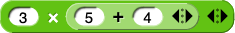you can see that the `+` block is an input to the `×` block, so the expression means 3×(5+4). Similarly,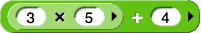means (3×5)+4. In Snap!, it's as if there are parentheses around every operation. But in text languages, you can write
`3 * 4 + 5`
without parentheses, so they need the rules you learn in math class (multiplication before addition, and so on). The `mod` operator is like multiplication and division; it happens before addition and subtraction. So for example,
`7 + 5 MOD 2 - 6`
means
`7 + 1 - 6`
, which is, of course, 2.
In a later lab, you can use your `even?` block to draw a brick wall because the even and odd numbered rows are different.1. Use `mod` to build a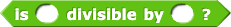predicate that tests for divisibility.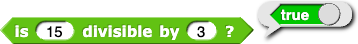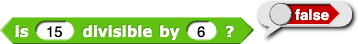2. Use this `divisible by?` predicate to build a predicate that tests whether its input is even (divisible by 2).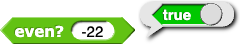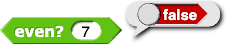3.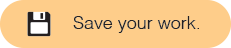AAP-2.H.2
The procedures
`move()`
and
`turn_clockwise()`
aren't built in to the AP's language so they are written in lower case like other programmer-defined procedures.
The conditional expression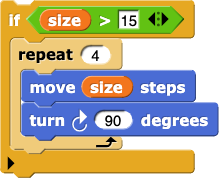would be written as
```IF(size > 15)
{
REPEAT 4 TIMES
{
move(size)
turn_clockwise(90)
}
}```
or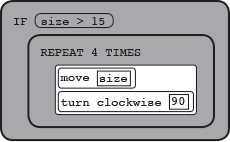As in Snap!, if the condition `(`size`) > 15` is `true`, the code inside the `if` statement runs; if the condition is `false`, the code does not run.

1. AAP-2.L.2
Algorithms that look the same may have different results. The following incomplete code fragment was designed to test if
`number`
is odd:
```IF (MISSING CONDITION)
{
DISPLAY "It is odd."
}```

Which of the following can be used in place of the MISSING CONDITION?
`number MOD 1 = 0`
`number MOD 1 = 1`
`number MOD 2 = 0`
`number MOD 2 = 1`
2. What's the value of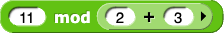?
1
0.2
2
2.2
3.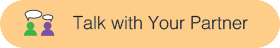Is it true that (12 MOD 2) > (11 MOD 2)? Explain your reasoning.
1. Build a predicate that tests whether its input is an integer. You may find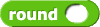useful.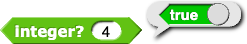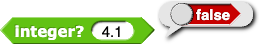Open your U2L3-Dots project, and save it again as U2L4-DotsTIF. Remind yourself about how the pictures are determined by the Boolean expression used.

1. Invent Boolean expressions for these pictures:
1.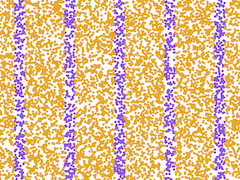2.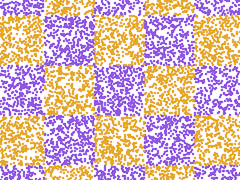3.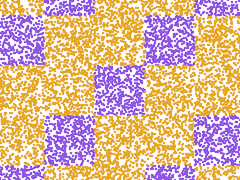4.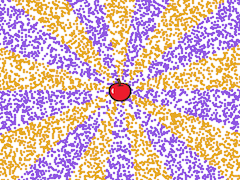5.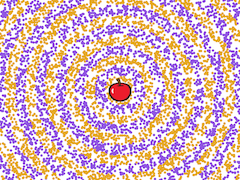6.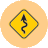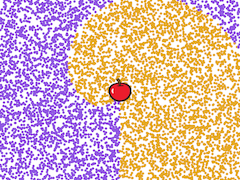Click for a hint.
Some of these include expressions of the form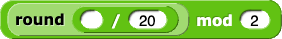with something like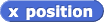in the empty input slot, and maybe different numbers in place of 20 and 2.